Winner: blessedx5
Prize: Sapphire Gemster Gift with Purchase

Win Free Webkinz Codes Now!

# Operation Gumball Basic Tutorial

With this strategy, you should be able to get to level 8 or 9 fairly reliably, and most of the time you can get through level 10, and work toward level 18 or 19. I developed this strategy after playing the game only for about a week. The advanced strategy that I use now is much more likely to get you past level 20, and perhaps to level 30. But, since it is more complicated, I have been struggling over the language to keep it as clear as possible, but there are a lot of exceptions, so I am just refining the language of my rules set.

OK, with that out of the way. Enjoy.

[...Continued...]

Operation Gumball is a classic logic game, based on a game that I grew up with – Master Mind.

In this game, you are given a set amount of guesses as to the hidden numbers, and on each response, you are given clues on whether the numbers you have are correct and in the right place.

So, if the number you are trying to guess is 190, and you put 123 as your first guess, you will see one green marker, to indicate that one of your numbers is correct and in the right place (the 1 is the first digit in the guess and the correct answer. In the same scenario, if your first guess was 012, you would receive a red ball, to indicate that one of your numbers is correct, but not in the right place (the 0 was the first digit of the guess, but the last digit of the correct answer).

My strategy for winning this game (and I have won a number of trophies on it, and several recently) is to first find a number that is not in the answer, and then use it to logically eliminate or place the correct numbers.

I have a number of screenshots that I will present and discuss the strategy. Although the screenshots might seem repetitive, they show the strategy in action (so, not just in theory.

First off, the game has 30 levels. Level 1 – 3 you have to guess a 3 digit number, with the answer not having any repeating digits (so, you will not see an answer like 112, because the number 1 can only appear one time in the answer). Level 4 – 6 you have to guess a 4 digit number. Level 6 – 9, you have to guess a 5 digit number. Level 10 you have to guess a 6 digit number. When you get to levels 10 – 20, you repeat the sequence, except you can have repeating digits (so 112 would be an acceptable answer, and 1114 would also be a correct answer). Levels 21 – 30 are really the same as 10 - 20, with repeating digits. You jut have less tries.

Later, I will fill in how you get points. I am still working on the exact way the game scores. For the most part, the scoring is very progressive (meaning that the higher you go, the greater the level scores will be). One example of the scoring is that if you answer the code in 6 tries on level 1, you get 1000 points, and if you do it on the 7th try, you get 950 (8th - 900 and so forth). The other levels also have similar differences - although I haven't documented all of them. Also, you get points for finding the numbers, and 2 reds equal a green. So, if you find a red on level 1, you get 100 points. If you find a green, you get 200 (or if you found a red and then found that same number as a green, you would get the 200 then). You can also get some small amounts by moving the red (so if you get a red on 144, and then try 414 and also get a red, you will get 100 for the first red and 2 for the second; if you then try 441 (with the 1 being the right number in the third digit), you will have 202 points for that one).

The scoring can be confusing - and really not worth going into in depth.

Of all of the games I have played, this is one of the few that I haven’t concerned myself with scoring because I just try to get through the levels as quick as I can – so I don’t know how understanding the scoring would improve my scoring – although it could hold a secret to how to win the trophy, which is assigned on some sort of random key.

So, my strategy is simple. Never introduce more than 2 unknown numbers in your guess. If you introduce 2 unknown numbers and you get the indication that one is part of the answer, you can easily tell which one it is on the very next move. If you were to introduce 3 numbers and 2 of them are correct, it could take 3 additional turns to figure out which is the correct one, and an additional turn to figure out its place.

When I begin the game, I am looking for a number that is not part of the answer, so that I can do the elimination.

So, I start with a sequence of 121. The reason that I do 121 instead of 112 is just ergonomic. It is easier to repeat the numbers like that, and when I get to later levels, it just takes a little less thinking. I used to do 112 on the first levels and 1122 – 11122 – 111222 (which is not wrong), but I found that doing the 121, 1212, 12121, 121212 was just easier on the fingers and the brain.
So, I do 121. If one of the numbers appears, I do 343. Suppose that none of those numbers are in the answer, I now know that 3 and 4 are numbers I can use to fill in the blanks (and will isolate the numbers I want to test. So, if the 121 produced a green ball, I will try 133. I know that the threes are “throwaways”, so all I am asking is if the 1 is correct in the first place. If I get a green ball, it obviously is. If I get a red ball, then the 1 is now the 3rd number _ _ 1. I know it is not the second number because in the first guess, when I did 121, I got a green, which means that the number that was correct in my guess is correct in its place.

So, instead of just using words to describe the techniques, take a look at these pictures. I am hoping that the range of pictures (while a bit repetitive), shows as many possible combinations.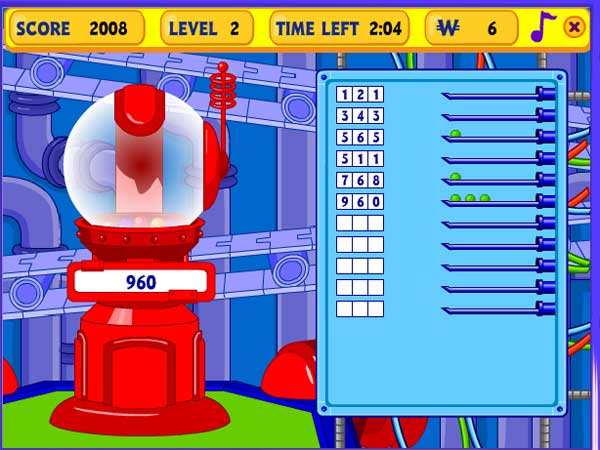In this case, you can see that my first 2 guesses produced no results. So, automatically, I know that I can use the number 1 as my elimination number. When 565 produced a green, I tried 511 – just to see if the 5 was the correct number, and n the correct place. When I found that 5 was not the right number, I knew it had to be 6 and it had to be the middle digit. In my next guess, I put the 6 in the middle, and continued with my sequence, with a 768. When this showed that only the 6 was correct, I knew that the final answer had to be 960 or 069 (since 9 and 0 were the only two numbers left in the sequence). You can see that there were still a number of tries left in this level.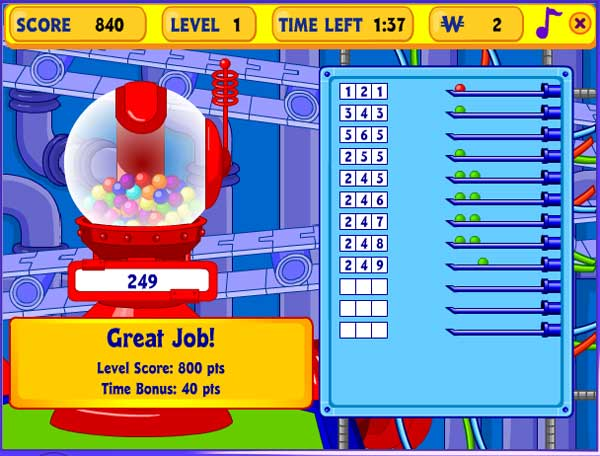In this trial, the first 121 resulted in a red ball. Since I don’t have an elimination number yet, I normally go ahead and try the next sequence of 2, so I put 343. When that turned up a green, I noted it, but still do not have an elimination number. So, I did 565, which showed that the 5 and 6 were numbers I could use to eliminate the 1 or the 2 (as well as the 3 or the 4).

Normally, when I have a red number in the first series, I put the 2nd number first, and then use my elimination number. So, I put 255, which showed that the 2 was correct in the first position.
After I got that, I proceeded to look at the second number (the 3 or 4). Again, using my known number (the 2) and my elimination number (5), I put 245. Since that showed that the 4 was correct, I knew the last digit was either 7, 8, 9, or 0. Note, I accidentally (or lazily) tried the 6 again, even though that was not correct. In this case, I did not get penalized for it since I had plenty of extra tries left.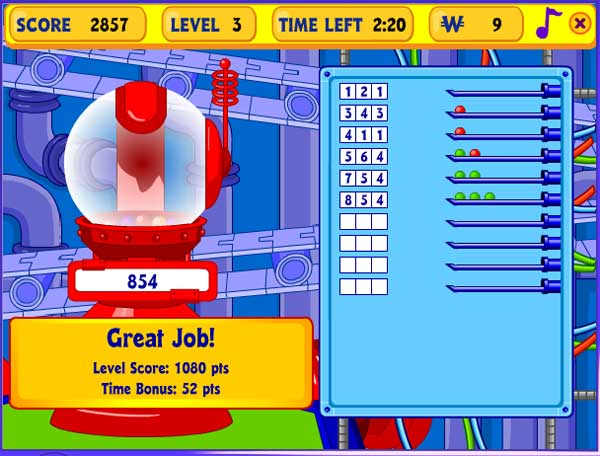Once again, I tried 121 and got no result. So, when I found that 343 produced a red, I used my rule of putting the 2nd number first and then the elimination numbers (411). Since this produced a red ball, I knew that the 4 was the correct number, and since it wasn’t supposed to be in the first digit, it had to be the last digit (since 343 produced a red, 4 could not be in the middle). So, I continued my sequence.

As I have said, I never put more than 2 unknown numbers in the next guess, and I typically go in numerical order, the next two numbers to try were the 5 and 6. Since I got a red, I knew that either the 5 or 6 were right, but they were in the wrong position. When I get down to only needing to guess 2 more numbers (which comes more into play in the later levels), I then reposition one of the numbers (so, I moved the 5 from the first digit to the second digit) and then try the next number in the sequence. So, 754 getting 2 greens tells me that the 5 is correct in the second digit. If I had gotten a red ball on 754, I would have known that the 5 was not right, and that the 7 should be in the middle (and by elimination, the 6 should be the first number).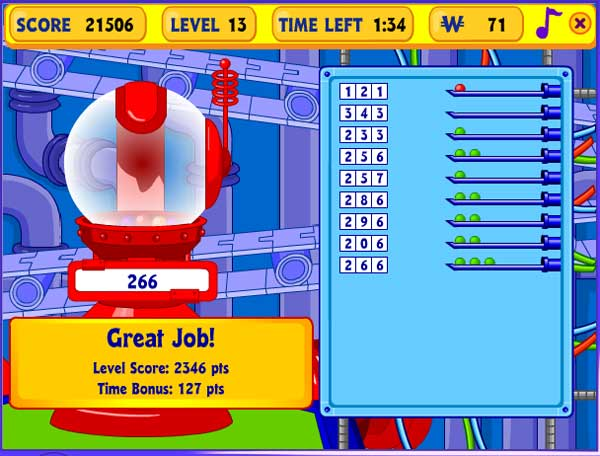When you get to levels above 10, as I mentioned before, you might get a number that appears in the answer twice (like above is 266). I don’t really intend to extend my tutorial too much past the first set of levels, but I do have some examples. For the most part, the strategy is the same. It is just more likely that you will have to make a guess near the last try.

If you look at the strategy, it is the same as before, except when I got all the way through the sequence, and still had only 2 green balls, I knew the other number had to be a 2 or a 6. I also knew it couldn’t be a 2, because I was trying to fill in the middle number (and in the first guess, if the middle were a 2, it would have been green). You can also see in the middle of the sequence, when the 56 reproduced a green, I did not isolate the 5 or 6 (mainly because I don’t have as many tries on level 13 as I would on level 11 or level 1), so I just tried the next number in sequence (257), and when that showed that the 5 was not correct, I knew the last digit was a 6).

OK, on to levels 4 – 6 (4 digits).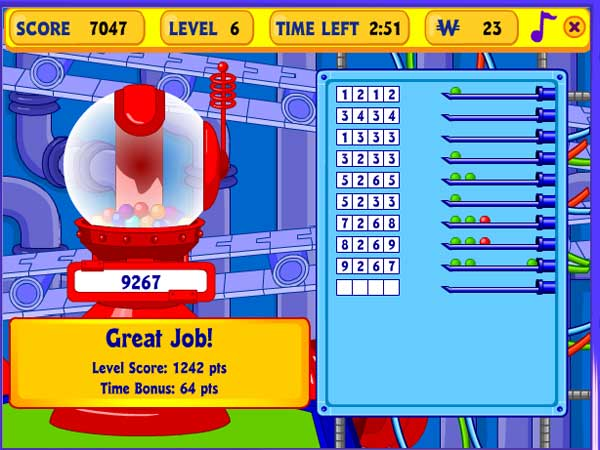Again, this uses the same principles. I start with a 1212 sequence, continuing my pattern until I find a number to use as an elimination number.

In this case, when I find that the 3434 produces no results, I decide the use the 3. When I get a green on the first try, I usually put the first number first and follow it with the elimination number. When 1333 does not produce an answer, I know that the 2 is the right number, and testing it with 3233 shows that it is in the second position (if that had been red, I would have known that the 2 was in the 4th position, since in the first try, I got a green).

Then I start my sequence again, adding 2 unknown numbers to my next try. Note: this screenshot reflects a technique where I fill in all of the unknown places with one of the 2 unknown numbers. In some screenshots, I only add 2 numbers and then fill in the remaining spots with elimination numbers. I normally tend to use the technique in this shot (but I wanted to acknowledge that there are some inconsistencies in my strategy).

When the 5256 reveals a green, I used my elimination number to figure which was correct (the 6 or the 5). By putting it in the first position, I will find out if the 5 is the correct number and if it is in the right position. If I get a green, I know the 5 is in the first digit. If I get a red, I know the 5 is in the last position (because of the earlier try). If I get not ball, I know that the 6 is in the third position. (which was the case here).

Finally, fill in the last 2 positions using the same technique I described earlier. When 726 8 reveals a red, I move one of the numbers (the 8 from the last digit to the first) and then add a new number (the 9). Since that also gives me a red, I now know that the 9 is correct and the 7 is correct in the last position.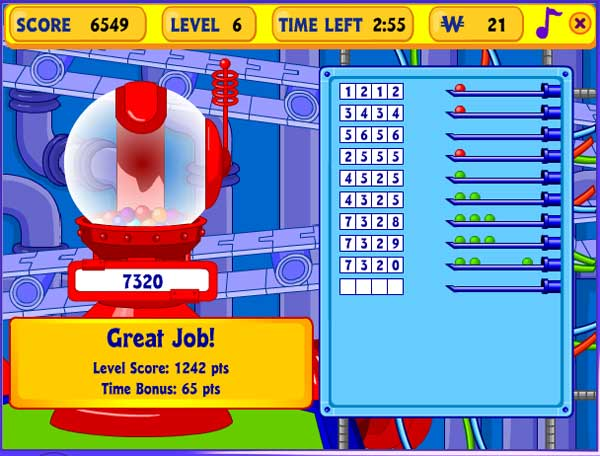I start off with a familiar pattern, adding numbers until I get an elimination number. As I mentioned before, if I have a red as my first try, I put the second number first, followed by the elimination numbers. When that reveals a red, I now know that the 2 should be in the 3rd digit.
I then repeat the procedure with the 3 and 4, putting the second number first and using the elimination numbers in the remaining unknown slots. I actually made a mistake here (which didn’t hurt me) when I put 4325. I ordinarily would have put 5325. It didn’t matter, because the try before showed that the 4 was not part of the sequence and could be used as an elimination number if I wanted. Finally, I got the last 2 numbers in the same manner I have described before with getting the final two numbers.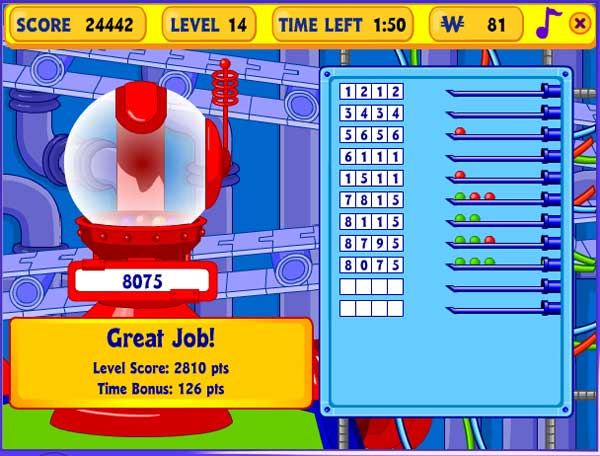Here, the 1212 and 3434 did not produce results, so I could use any of those as elimination numbers. When 6111 did not produce a result, I knew the 5 was correct, and it had to be in either the 2nd or 4th position. When I put it in the 2nd position and it returned a red, it had to be in the 4th position. So, I could continue with my sequence, using the 7 / 8 next. Here, I filled in the 3rd digit with a 1 (my elimination number). This is contrary to how I did it earlier – where I mentioned a different technique. On the whole, it is probably better to not use this technique (I should have put 7875 in that guess. But, you can look at how I filled in the other numbers and see the pattern.Finally, here is one on the harder level. When I saw 1212 received a green and red, and then later on when I saw that 1777 (using my elimination number) returned nothing, I knew there were two 2’s in the answer.

OK. On to the next level. Up to level 7, I can get every single time. I can usually get to level 10 without any problem but levels 7-9 will occasionally cause problems (normally if there is a 0 and 9 in the number, just because of how my elimination sequences go).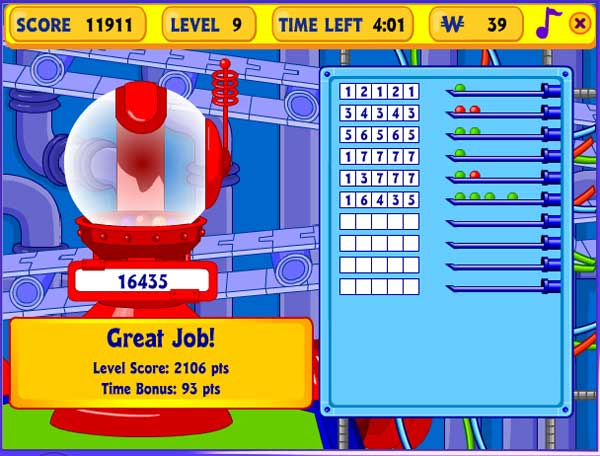Here you can see that I use the same principles. I start with 121212, and keep going until I get an elimination number. In this case, the elimination number came when, after the third guess, I had 5 balls already. So, I knew that 7,8,9 and 0 were all eliminated.

This one looks like a quick guess to get to the final, but it is using the same logic. After finding out the 1 was correct, and that the 3 was not correct, I knew that the 1 went in the first digit and the 3 went in the fourth digit. Since the 6 and 5 were correct (2 greens) and the 4 and 3 were incorrect, I knew the 4 had to go in the middle spot or end spot. I also knew that the 6 could only go in the second digit. So, I knew the answer was either 16435 or 16534. It turned out it was the first one.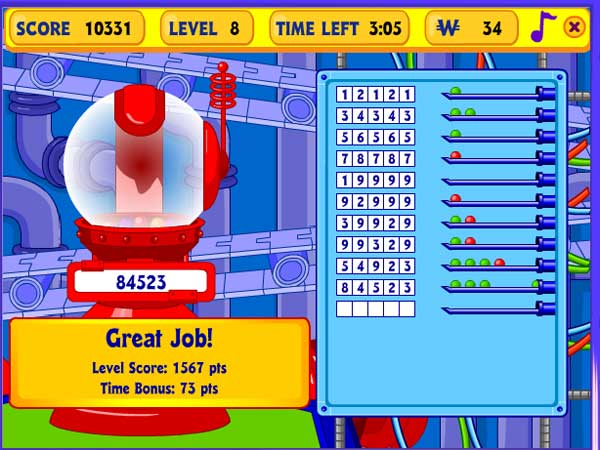This shows a similar scenario. After 4 guesses, I had 5 balls, so 9 and 0 were eliminated. I tested the one first (since I do that when I get a green), and then tested the 2, finding that the 2 went in the 4th digit. Then, I systematically tested the 3, 5/6 and 78 to get the results.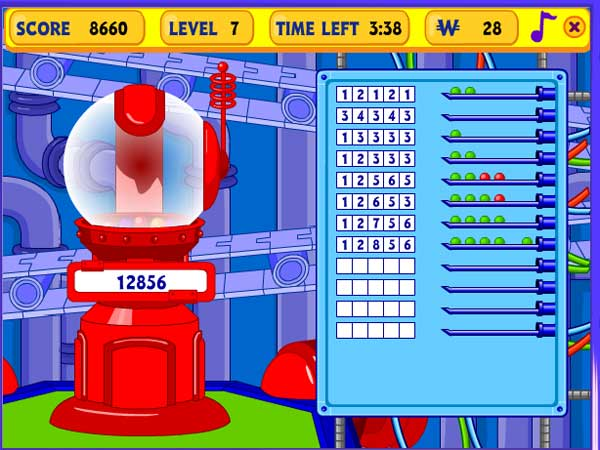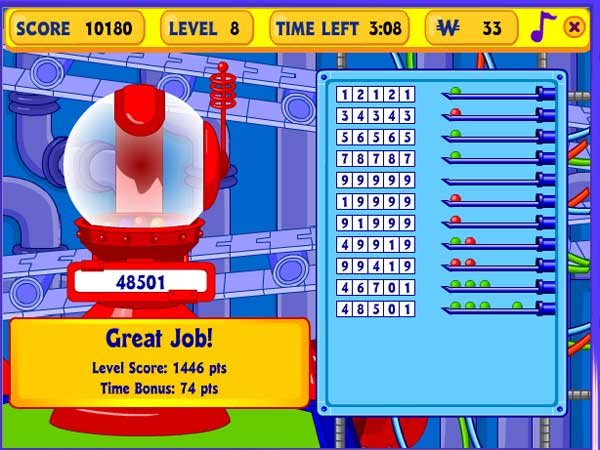This is one of the harder scenarios – and one of the most likely to not get through. After 4 tries, I only had 4 numbers. If I had done 909090, I would have gotten the 5th, but not known which. I did not have an elimination number yet. So, I just did all 9s, to eliminate either the 9 or the 0. Then I tested the numbers, and probably guessed a little luckily or else I wouldn’t have made it through (If I had tried the 3 and / or the 6, I would have run out of guesses.
Finally, onto level 10. Here is where I sometimes stray from my logic and have to take a different route (although sometimes I can get through with my strategy). I don’t have a screenshot showing a 121212 strategy – I will get one when I get around to it. Instead, I use a different technique.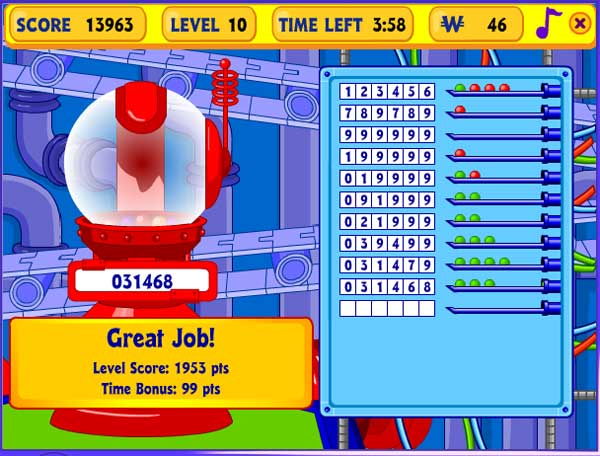By doing 123456 and 789789, by the 3rd try, I know can eliminate a number. In this case, I was able to eliminate the 9. Then, you can see the process I used to identify which of the 4 numbers in the first try are correct.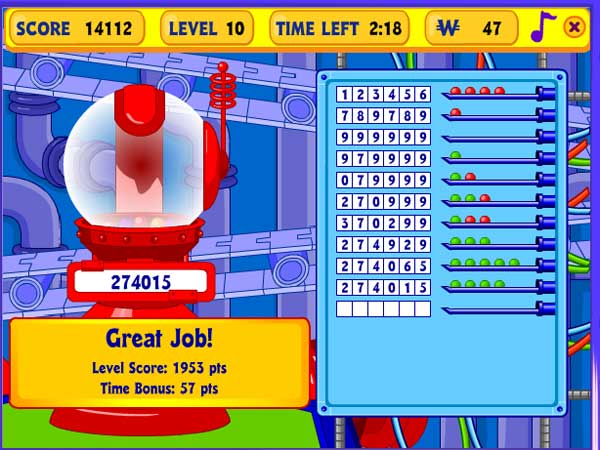Again, this produced a similar result. You can see here where I try to eliminate multiple numbers at a time by shifting them to the right, and then comparing the results. I got lucky with the 7 coming up when it did. I also got lucky by having all reds in the first 2 (because that eliminates all of those numbers in that position – if there was a single green, it is just harder).

The shift technique involves starting out with one test (079999 – I knew the 7 was good and the 9s are elimination). Then, I tried 270999. I shifted the 0 to the third position and added a 2. After that, I kept the 0 stable, added a 3, and moved the 2 to the 4th position. When I got the same number of balls (3), but one less green, I knew the 2 went in the first position. Then I actually made a mistake (I meant to shift the zero again when I added the 4 – but it worked out OK). Finally, I put the 6 and 5 in there (but in positions opposite at the start because of the all reds in the start. I knew I had 2 more tries to figure out if I had to add the 1 and in which position it had to be (because it could just have easily been 275061).

OK. There it is. A lot of explanation, a lot of screenshots, and hopefully a lot of Kinzcash. If you win a bunch of trophies and want to send me one as a way of gratitude, I think that would be great. Otherwise, enjoy.

Let me add one more amendment to this. The first change that I made to my strategy was that, starting on level 4, instead of using 1212, 3434, 5656, 7878, I used 1212, 3344, 5665. This offset some of the numbers and made it a bit easier to deduce which possibilities the final answer could have. All of the other principles are the same -- I just don't have any screenshots for that because I advanced my strategy to the next level before I started working on another tutorial.

So, enjoy, and stay tuned for the advanced strategy later in the summer.